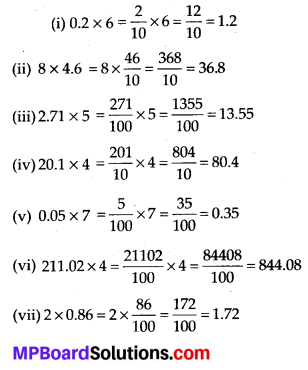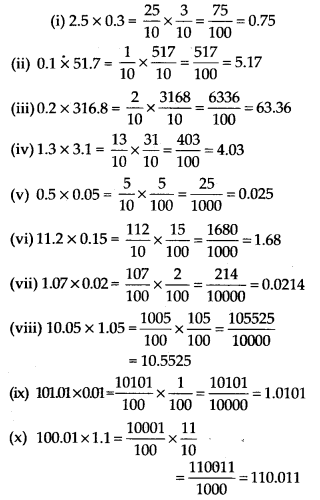# MP Board Class 7th Maths Solutions Chapter 2 Fractions and Decimals Ex 2.6

## MP Board Class 7th Maths Solutions Chapter 2 Fractions and Decimals Ex 2.6

Question 1.
Find:
(i) 0.2 × 6
(ii) 8 × 4.6
(iii) 2.71 × 5
(iv) 20.1 × 4
(v) 0.05 × 7
(vi) 211.02 × 4
(vii) 2 × 0.86
Solution:Question 2.
Find the area of rectangle whose length is 5.7 cm and breadth is 3 cm.
Solution:
Length = 5.7 cm and breadth = 3 cm
Area = Length × Breadth = (5.7 × 3) cm2 = 17.1 cm2Question 3.
Find:
(i) 1.3 × 10
(ii) 36.8 × 10
(iii) 153.7 × 10
(iv) 168.07 × 10
(v) 31.1 × 100
(vi) 156.1 × 100
(vii) 3.62 × 100
(viii) 43.07 × 100
(ix) 0.5 × 10
(x) 0.08 × 10
(xi) 0.9 × 100
(xii) 0.03 × 1000
Solution:
We know that when a decimal number is multiplied by 10, 100, 1000, the decimal point in the product is shifted to the right by as many places as there are zeros.
(i) 1.3 × 10 = 13
(ii) 36.8 × 10 = 368
(iii) 153.7 × 10 = 1537
(iv) 168.07 × 10 = 1680.7
(v) 31.1 × 100 = 3110
(vi) 156.1 × 100 = 15610
(vii) 3.62 × 100 = 362
(viii) 43.07 × 100 = 4307
(ix) 0.5 × 10 = 5
(x) 0.08 × 10 = 0.8
(xi) 0.9 × 100 = 90
(xii) 0.03 × 1000 = 30

Question 4.
A two-wheeler covers a distance of 55.3 km in one litre of petrol. How much distance will it cover in 10 litres of petrol?
Solution:
Distance covered in 1 litre of petrol = 55.3 km
Distance covered in 10 litres of petrol = (10 × 55.3) km = 553 kmQuestion 5.
Find:
(i) 2.5 × 0.3
(ii) 0.1 × 51.7
(iii) 0.2 × 316.8
(iv) 1.3 × 3.1
(v) 0.5 × 0.05
(vii) 1.07 × 0.02
(viii) 10.05 × 1.05
(ix) 101.01 × 0.01
(x) 100.01 × 1.1
Solution: